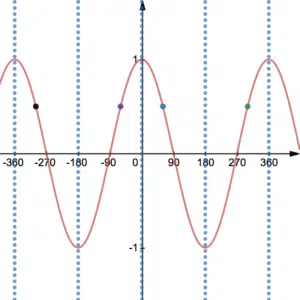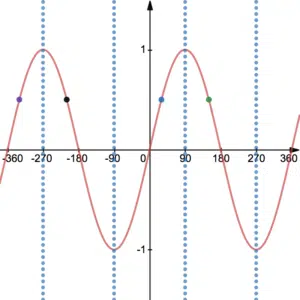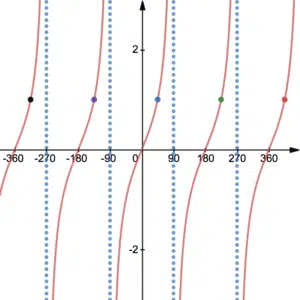# Trigonometric Equations

Trigonometric equations can be solved by first manipulating them into the form

$\sin(x)=a$,   $\cos(y)=b$   or   $\tan(z)=c$.

This could be very simple, a straight forward manipulation of a linear equation (Examples 1 and 2) or it could be a more complicated manipulation of a quadratic equation (Examples 3 and 4). Once in this format, one can use the $\sin^{-1}$, $\cos^{-1}$ and $\tan^{-1}$ buttons on a scientific calculator. However, at this stage it is very important to capture all solutions of the trigonometric equation since the scientific calculator will only give one default solution.

Note that when factorising a trigonometric quadratic, some factors do not produce solutions. For example, $\cos(x)=2$ does not have any solutions. This is because sin and cos only takes values between -1 and 1. Tan, however, is not restricted in this way but does have asymptotes where solutions are restricted – see the tan graph above (Example 1). In both cases above (Examples 3 and 4), both factors produced solutions.

## Solving trigonometric equations using the trigonometric graphs

Once in the form described above, a calculator will only give you a default solution, it is up to you to find the rest. Remind yourselves of the trigonometric graphs. The following rules can be used to find all solutions on a given interval:

$y=\cos(x):$The local lines of symmetry on the graph of $\cos(x)$ are every $180^\circ$ starting at $0^\circ$. If there is a solution at $60^\circ$, for example, there is also one at $360-60=300^\circ$. Both of these solutions then repeat every $360^\circ$.

$y=\sin(x):$The local lines of symmetry on the graph of $\sin(x)$ are every $180^\circ$ starting at $90^\circ$. If there is a solution at $30^\circ$, for example, there is also one at $180-30=150^\circ$. Both of these solutions then repeat every $360^\circ$.

$y=\tan(x):$The solutions on the tan graph are easier to find, the default value simply repeats every $180^\circ$. If there is a solution at $45^\circ$, for example, there is another at $-135^\circ$, $225^\circ$, etc.

The tan graph is different to the sin and cos graphs. Firstly, tan can take a value between $-\infty$ and $\infty$ whereas sin and cos take values between -1 and 1. The graph of tan also has asymptotes as a result of dividing by $\cos(x)=0$ in the expression $\tan(x)=\frac{\sin(x)}{\cos(x)}$.

## A note on 'CAST'

It may be that the argument of the trigonometric function is a function of $x$. In these cases it is very important to extend the interval of solution values so that the full set of solutions are obtained. See Examples 2 and 4. Some students prefer to use ‘CAST’ – this is a complicated method for finding all of the solutions of trigonometric equations. See ‘CAST’ on YouTube. It is considerably simpler, as you will see in the examples below, to extend the interval of solutions according to the operations performed on $x$.

## Examples of Trigonometric Equations

1. Solve $\sqrt{2}\sin(x)=\cos(x)$ on the interval $-360^\circ\leq x\leq 360^\circ$.
2. Solve $\tan(x+75^\circ)=3$ on the interval $0^\circ\leq x\leq 360^\circ$.

Solve the following for $-180^\circ\leq x\leq 180^\circ$:

$4\cos(2x)-5=-1$

Solve the following for $0\leq x\leq 360^\circ$:

$\sin^2(x)=\sin(x)$

Solve

$6\sin^2(3\theta)=4-\cos(3\theta)$

on the interval $0<\theta<180^\circ$.